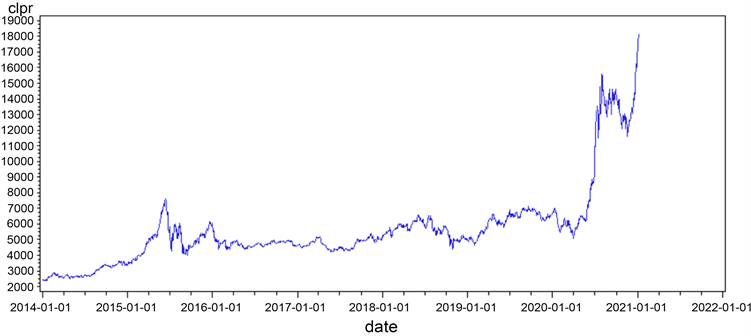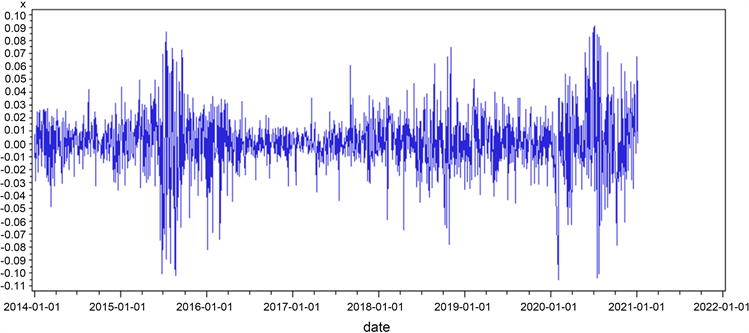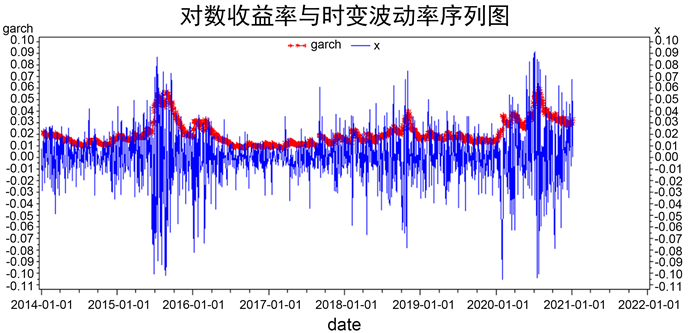#### 期刊菜单

Study on the Impact of COVID-19 Epidemic on Chinese Tourism Industry
DOI: 10.12677/SA.2022.112044, PDF, HTML, XML, 下载: 93  浏览: 213

Abstract: The COVID-19 epidemic has brought a great impact on China’s tourism industry. This paper analyzes the development of China’s tourism industry in the past seven years based on the daily data of 1,709 Shenwan Tourism Composite Index from January 2, 2014 to January 5, 2021. In addition, the TGARCH-M model with high fitting degree following the T-distribution state was constructed by integrating the fluctuating impact amount into the comprehensive index generation process of tourism industry during the epidemic period, and the additional impact of COVID-19 epidemic on China’s tourism industry was studied. The empirical results show that the fluctuation of China’s tourism industry in the market in the recent seven years shows a positive feedback effect, and the positive impact has no obvious “leverage” effect compared with the negative impact. After the outbreak of the epidemic, the Fluctuating impact of tourism index increased by 2.08 × 10−5 units. Due to the small impact value of additional fluctuations, it indicates that the impact of the epidemic on China's tourism industry is not so serious, and it also indicates that the tourism industry can recover at a fast speed after the epidemic.

1. 引言

2020年的春节前后，突然爆发的新冠肺炎疫情给我国的经济社会与人民生活带来了较大冲击。在一年多的疫情期间，中国采取了严格隔离的防控措施，包括封闭城市、边境、限制交通工具出行等，以遏制“新冠肺炎”疫情的传播(王箐等，2020) 。事实证明这些措施是有效的，有效地控制住了疫情的恶化。但是这些举措也对我国经济产生了重要影响，如消费减少，产出下降；短期物价上升，失业率上升；金融机构风险增加，产业发展遭受巨大损失等(何诚颖等，2020) 。作为一切都建立在以“流动性”特点经营的旅游行业，更遭受了巨大的冲击影响。新冠肺炎疫情可谓为中国的旅游业带来了暂停式的冲击(刘淑芳和杨虎涛，2020) 。如今，已有很多学者对我国疫情下的旅游行业进行了深入研究。明庆忠与赵建平(2020)  分析了新冠肺炎疫情影响旅游业的发展机制、特征、表现与后果，并针对本次新冠肺炎疫情防控进程提出相应的管理要求，认为旅游企业、政府与行业协会三方应该共同合作，形成合作共同体以共克时艰。王少华(2020)  对在疫情下的河南省旅游业的冲击表征与影响机理进行了研究，他认为应该正确看待疫情之下旅游业的“危”与“机”。在这严峻的形势下，加强疫情对旅游业冲击波动的系统评估与科学预测，并提出一些有效的恢复旅游业的纾困措施，相信这是学者们当今研究的必然方向(申军波等，2020) 。

2. 模型构建与修正

2.1. GARCH族模型介绍

${x}_{t}={\phi }_{0}+{\phi }_{1}{x}_{t-1}+\cdots +{\phi }_{i}{x}_{t-i}+{\epsilon }_{t}$ (1)

${\epsilon }_{t}={\sigma }_{t}{v}_{t}$ (2)

${\sigma }_{t}^{2}={\beta }_{1}{\sigma }_{t-1}^{2}+{\beta }_{2}{\sigma }_{t-2}^{2}+\cdots +{\beta }_{p}{\sigma }_{t-p}^{2}+{\alpha }_{0}+{\alpha }_{1}{\epsilon }_{t-1}^{2}+\cdots +{\alpha }_{q}{\epsilon }_{t-q}^{2}$ (3)

(3)式中参数具有非负约束，即 ${\alpha }_{i}\ge 0,i=1,2,\cdots ,q;{\beta }_{j}\ge 0,j=1,2,\cdots ,p$，且模型中 ${v}_{t}$ 独立同分布， $E\left({v}_{t}\right)=0,Var\left({v}_{t}\right)=1$。模型中p与q分别表示GARCH项与ARCH的阶数，系数 ${\alpha }_{i}$ 代表市场新消息为波动带来的影响， ${\beta }_{j}$ 代表旧的消息为市场波动带来的影响。

${\sigma }_{t}^{2}={\beta }_{1}{\sigma }_{t-1}^{2}+{\alpha }_{0}+{\alpha }_{1}{\epsilon }_{t-1}^{2}+\gamma {\epsilon }_{t-1}^{2}{d}_{t-1}$ (4)

TGARCH模型是用来解释当信息存在非对称效应时，利用虚拟变量来区分坏的信息与好的信息对收益率波动的影响：当 ${\epsilon }_{t-1}<0$ 时，及坏的信息冲击时， ${d}_{t-1}=1$ ；否则 ${d}_{t-1}=0$$\gamma {\epsilon }_{t-1}^{2}{d}_{t-1}$ 称为TARCH项，也称为非对称效应项，当 $\gamma \ne 0$ 时就认为存在着非对称性。易证明当序列受到“坏的信息”冲击时其波动性为 ${\alpha }_{1}+\gamma$ 倍，受到“好的信息”冲击时其波动减小为 ${\alpha }_{1}$ 倍。

${x}_{t}={\phi }_{0}+{\phi }_{1}{x}_{t-1}+\cdots +{\phi }_{i}{x}_{t-i}+{\epsilon }_{t}+\delta {\sigma }_{t}^{2}$ (5)

${x}_{t}={\phi }_{0}+{\phi }_{1}{x}_{t-1}+\cdots +{\phi }_{i}{x}_{t-i}+{\epsilon }_{t}+\delta {\sigma }_{t}$ (6)

${x}_{t}={\phi }_{0}+{\phi }_{1}{x}_{t-1}+\cdots +{\phi }_{i}{x}_{t-i}+{\epsilon }_{t}+\delta \mathrm{ln}\left({\sigma }_{t}^{2}\right)$ (7)

2.2. 模型修正

${x}_{t}={\phi }_{0}+{\phi }_{1}{x}_{t-1}+\cdots +{\phi }_{i}{x}_{t-i}+{\epsilon }_{t}+\delta {\sigma }_{t}$ (8)

${W}_{t}=\left\{\begin{array}{l}0,\text{\hspace{0.17em}}\text{\hspace{0.17em}}t<2020\text{ }\text{ }年\text{ }\text{ }1\text{ }\text{ }月\text{ }\text{ }1\text{ }\text{ }日\\ 1,\text{\hspace{0.17em}}\text{ }\text{\hspace{0.17em}}t\ge 2020\text{ }\text{ }年\text{ }\text{ }1\text{ }\text{ }月\text{ }\text{ }1\text{ }\text{ }日\end{array}$ (9)

${\sigma }_{t}^{2}={\beta }_{1}{\sigma }_{t-1}^{2}+{\alpha }_{0}+{\alpha }_{1}{\epsilon }_{t-1}^{2}+\gamma {\epsilon }_{t-1}^{2}{d}_{t-1}+\rho {W}_{t}$ (10)

3. 数据分析

3.1. 数据选取与处理

3.2. 描述性统计分析Figure 1. Timing chart of closing price of Shenwan tourism composite indexFigure 2. Timing chart of Shenwan tourism comprehensive index yieldTable 1. Descriptive statistical analysis of daily logarithm return rate of Shenwan tourism comprehensive index

4. 实证结果与分析

4.1. ARCH效应检验

${x}_{t}=0.0011775-0.06824{x}_{t-2}+0.08527{x}_{t-7}+{\epsilon }_{t}$ (11)Table 2. Residual test of ARCH effect after regression equation

4.2. 基于TGARCH-M模型的实证分析

${x}_{t}=-0.0663{x}_{t-2}+0.0553{x}_{t-7}+{\epsilon }_{t}+0.0874{\sigma }_{t}$ (12)

${\sigma }_{t}^{2}=0.9229{\sigma }_{t-1}^{2}+2.4144×{10}^{-6}+0.0794{\epsilon }_{t-1}^{2}-0.0131{\epsilon }_{t-1}^{2}{d}_{t-1}+2.08×{10}^{-5}{W}_{t}$ (13)Table 3. TGARCH(1,1)-M model parameter estimation注：浅色线代表对数收益率时序图，深色线代表波动率序列时序图；横坐标的每一小格代表一个季度。

Figure 3. Sequence diagram of log return and time-varying volatility of Shenwan tourism comprehensive index

5. 总结

  王箐, 王钟黎, 李士雪, 薛付忠. “新冠肺炎”疫情对中国股市价格波动的短期影响[J]. 经济与管理评论, 2020, 36(6): 16-27.  何诚颖, 闻岳春, 常雅丽, 耿晓旭. 新冠病毒肺炎疫情对中国经济影响的测度分析[J]. 数量经济技术经济研究, 2020, 37(5): 3-22.  刘淑芳, 杨虎涛. 新冠疫情对中日韩三国旅游业的影响研究[J]. 亚太经济, 2020(2): 21-29.  明庆忠, 赵建平. 新冠肺炎疫情对旅游业的影响及应对策略[J]. 学术探索, 2020(3): 124-131.  王少华, 王璐, 王梦茵, 王伟. 新冠肺炎疫情对河南省旅游业的冲击表征及影响机理研究[J]. 地域研究与开发, 2020, 39(2): 1-7.  申军波, 徐彤, 陆明明, 翟燕霞. 疫情冲击下旅游业应对策略与后疫情时期发展趋势[J]. 宏观经济管理, 2020(8): 55-60.  严伟, 严思平. 新冠疫情对旅游业发展的影响与应对策略[J]. 商业经济研究, 2020(11): 190-192.  王庆生, 刘诗涵. 新冠肺炎疫情对国内游客旅游意愿与行为的影响[J]. 地域研究与开发, 2020, 39(4): 1-5.  王金伟, 王国权, 王欣, 张俊娇. 新冠肺炎疫情下公众焦虑心理对出游意愿的影响研究——旅游业恢复信心的中介作用[J]. 西南民族大学学报(人文社会科学版), 2020, 41(11): 220-227.  孙玉环. 基于SARIMA模型的SARS对中国入境旅游收入影响的定量分析[J]. 旅游科学, 2006(1): 18-21.  俞金国, 王丽华. 后SARS时期中国入境旅游人数恢复评估——基于ARIMA模型[J]. 统计与决策, 2005(14): 84-87.  朱迎波, 葛全胜, 魏小安, 董培南, 何连生, 彭唬. SARS对中国入境旅游人数影响的研究[J]. 地理研究, 2003, 22(5): 551-559.  桂文林, 韩兆洲. 危机事件对中国入境旅游外汇收入影响评估[J]. 旅游学刊, 2010, 25(12): 28-36.  Engle, R. (1982) Autoregressive Conditional Heteroskedasticity with Estimates of the Variance of U.K. Inflation. Econometrica, 50, 987-1008. https://doi.org/10.2307/1912773  Bollerslev, T. (1986) Generalized Autoregressive Conditional Heteroskedasticity. Journal of Econometrics, 31, 307-327. https://doi.org/10.1016/0304-4076(86)90063-1  Glosten, L.R., Jagannathan, R. and Runkle, D.E. (1993) On the Relation between the Expected Value and the Volatility of the Nominal Excess Return on Stocks. Journal of Finance, 48, 1779-1801. https://doi.org/10.1111/j.1540-6261.1993.tb05128.x  Robert, E., Lilien, D.M. and Robins, R.P. (1987) Estimating Time Varying Risk Premia in the Term Structure: The ARCH-M Model. Econometrica, 55, 391-407. https://doi.org/10.2307/1913242